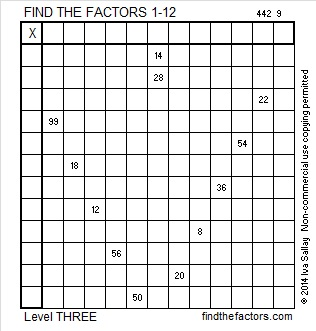# 271 and Level 3

• 271 is a prime number.
• Prime factorization: 271 is prime.
• The exponent of prime number 271 is 1. Adding 1 to that exponent we get (1 + 1) = 2. Therefore 271 has exactly 2 factors.
• Factors of 271: 1, 271
• Factor pairs: 271 = 1 x 271
• 271 has no square factors that allow its square root to be simplified. √271 ≈ 16.462How do we know that 271 is a prime number? If 271 were not a prime number, then it would be divisible by at least one prime number less than or equal to √271 ≈ 16.462. Since 271 cannot be divided evenly by 2, 3, 5, 7, 11, or 13, we know that 271 is a prime number.

Here is today’s factoring puzzle:Print the puzzles or type the factors on this excel file: 12 Factors 2014-10-20

A Logical Approach to FIND THE FACTORS: Find the column or row with two clues and find their common factor. Write the corresponding factors in the factor column (1st column) and factor row (top row).  Because this is a level three puzzle, you have now written a factor at the top of the factor column. Continue to work from the top of the factor column to the bottom, finding factors and filling in the factor column and the factor row one cell at a time as you go.